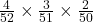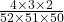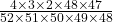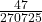## Score on last try: 0 of 8 pts. See Details for more. Get a similar question You can retry this question below Five cards a

Question

Score on last try: 0 of 8 pts. See Details for more.
Get a similar question
You can retry this question below
Five cards are drawn randomly from a standard deck of 52 cards
Determine the probability that exactly 3 of these cards are Aces. Write your answer in decimal form, rounded to 5 decimal
places

in progress 0
2 months 2021-09-02T15:27:16+00:00 1 Answers 0 views 0

there are 4 aces in the deck of 52 cards

so the probability to draw one ace is 4/52

if we draw an ace, there are 3 left in 51 cards.

so the next probability will be 3/51

we need to multiply probabilities to know how likely it is that this happens in a row.

for three in a row that’srewritten its:we’ll see soon why this is easier to write.

we also have to account for the two cards that are no aces. it doesn’t matter if the first two cards are no aces, the last two, or any rodney. just the ammount of non-aces is important.

there are 48 non-aces in the deck.

so the probability to draw one of them in the first step is 48/52.

wich each draw the number of cards left in the deck decreases by 1. and depending what we draw, that ammount also decreases.

we draw 5 cards, so we know what the denominators will be. the numerator will be the rest-ammounts of each event, like shown in the complicated fraction above.

let’s write it most easily as this:this scenario looks like it only describes 3 aces, then two non-aces, but the order of multiplication isn’t important. you could put the 48 and 47 to the left, same solution. so this expression represents all permutations of “3 aces when drawing 5 cards”, including the two cards that are not aces.

that’s why writing it as one convoluted fraction is indeed better and simpler than many fraction multiplied in row.

time to grap a calculator.

our probability for the event is

54144 possible valid outcomes out of

311875200 possible combinations.

luckily the calculator can reduce that to a simpler form of(reduced with 2^6*3^, big number, but tried it out by hand stacking up prime factors until hittinga prime in the numerator)

written as a readable kind of number you probably know and like it’s

0.0001736079047

in decimal form. now rounded as asked for:

0.00017

(as percentage: 0.017%)

hope the way how we got here is somewhat clear. feel free to ask any questions.

kind regards

Alex